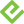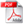# Decision Analytics: Microsoft Excel

### eBook (Watermarked)

• List Price: \$31.99
• Includes EPUB, MOBI, and PDF
• This eBook includes the following formats, accessible from your Account page after purchase:EPUB The open industry format known for its reflowable content and usability on supported mobile devices.MOBI The eBook format compatible with the Amazon Kindle and Amazon Kindle applications.PDF The popular standard, used most often with the free Adobe® Reader® software.

This eBook requires no passwords or activation to read. We customize your eBook by discreetly watermarking it with your name, making it uniquely yours.

## Description

• Dimensions: 7" x 9-1/8"
• Edition: 1st
• eBook (Watermarked)
• ISBN-10: 0-13-349059-9
• ISBN-13: 978-0-13-349059-6

Crunch Big Data to optimize marketing and more!

Overwhelmed by all the Big Data now available to you? Not sure what questions to ask or how to ask them? Using Microsoft Excel and proven decision analytics techniques, you can distill all that data into manageable sets—and use them to optimize a wide variety of business and investment decisions. In Decision Analytics: Microsoft Excel, best selling statistics expert and consultant Conrad Carlberg will show you how—hands-on and step-by-step.

Carlberg guides you through using decision analytics to segment customers (or anything else) into sensible and actionable groups and clusters. Next, you’ll learn practical ways to optimize a wide spectrum of decisions in business and beyond—from pricing to cross-selling, hiring to investments—even facial recognition software uses the techniques discussed in this book!

Through realistic examples, Carlberg helps you understand the techniques and assumptions that underlie decision analytics and use simple Excel charts to intuitively grasp the results. With this foundation in place, you can perform your own analyses in Excel and work with results produced by advanced stats packages such as SAS and SPSS.

This book comes with an extensive collection of downloadable Excel workbooks you can easily adapt to your own unique requirements, plus VBA code to streamline several of its most complex techniques.

• Classify data according to existing categories or naturally occurring clusters of predictor variables
• Cut massive numbers of variables and records down to size, so you can get the answers you really need
• Utilize cluster analysis to find patterns of similarity for market research and many other applications
• Learn how multiple discriminant analysis helps you classify cases
• Use MANOVA to decide whether groups differ on multivariate centroids
• Use principal components to explore data, find patterns, and identify latent factors

## Sample Content

Introduction     1

What’s in the Book     1

Why Use Excel?     3

1  Components of Decision Analytics     5

Classifying According to Existing Categories     5

Using a Two-Step Approach     6

Multiple Regression and Decision Analytics     6

Multivariate Analysis of Variance     9

Discriminant Function Analysis     10

Logistic Regression     12

Classifying According to Naturally Occurring Clusters      13

Principal Components Analysis     13

Cluster Analysis     14

Some Terminology Problems     16

The Design Sets the Terms     17

Causation Versus Prediction     18

Why the Terms Matter     18

2  Logistic Regression     21

The Rationale for Logistic Regression     22

The Scaling Problem     24

Equal Variances with Dichotomies     27

Equal Spread and the Range     28

The Distribution of the Residuals     29

Calculating the Residuals     30

The Residuals of a Dichotomy     30

Using Logistic Regression     31

Using Odds Rather Than Probabilities     32

Using Log Odds     33

Using Maximum Likelihood Instead of Least Squares     34

Maximizing the Log Likelihood     35

Setting Up the Data     35

Setting Up the Logistic Regression Equation     36

Getting the Odds     38

Getting the Probabilities     39

Calculating the Log Likelihood     40

Finding and Installing Solver     41

Running Solver     41

The Rationale for Log Likelihood     43

The Probability of a Correct Classification     44

Using the Log Likelihood     45

The Statistical Significance of the Log Likelihood     48

Setting Up the Reduced Model     50

Setting Up the Full Model     51

3  Univariate Analysis of Variance (ANOVA)     53

The Logic of ANOVA     54

Using Variance     54

Partitioning Variance     55

Expected Values of Variances (Within Groups)     56

Expected Values of Variances (Between Groups)     58

The F-Ratio     61

The Noncentral F Distribution     64

Single Factor ANOVA     66

Computing the Statistics     67

Deriving the Standard Error of the Mean     70

Using the Data Analysis Add-In     72

Installing the Data Analysis Add-In     73

Using the ANOVA: Single Factor Tool     73

Understanding the ANOVA Output     75

Using the Descriptive Statistics      75

Using the Inferential Statistics     76

The Regression Approach     79

Using Effect Coding     80

The LINEST() Formula     82

The LINEST() Results     83

LINEST() Inferential Statistics     85

4  Multivariate Analysis of Variance (MANOVA)     89

The Rationale for MANOVA     89

Correlated Variables     90

Correlated Variables in ANOVA     91

Visualizing Multivariate ANOVA     92

Univariate ANOVA Results     93

Multivariate ANOVA Results     93

Means and Centroids     95

From ANOVA to MANOVA     96

Using SSCP Instead of SS     98

Getting the Among and the Within SSCP Matrices     102

Sums of Squares and SSCP Matrices     104

Getting to a Multivariate F-Ratio     105

Wilks’ Lambda and the F-Ratio     107

Converting Wilks’ Lambda to an F Value     108

Running a MANOVA in Excel     110

Laying Out the Data     110

Running the MANOVA Code     111

Descriptive Statistics     112

Equality of the Dispersion Matrices     113

The Univariate and Multivariate F-Tests     115

After the Multivariate Test     116

5  Discriminant Function Analysis: The Basics     119

Treating a Category as a Number     120

The Rationale for Discriminant Analysis     122

Multiple Regression and Discriminant Analysis     122

Discriminant Analysis and Multiple Regression     125

Regression, Discriminant Analysis, and Canonical Correlation     125

Coding and Multiple Regression     127

The Discriminant Function and the Regression Equation     129

From Discriminant Weights to Regression Coefficients     130

Eigenstructures from Regression and Discriminant Analysis     133

Wrapping It Up     137

6  Discriminant Function Analysis: Further Issues     139

Using the Discriminant Workbook     139

Opening the Discriminant Workbook     140

Using the Discriminant Dialog Box     141

Why Run a Discriminant Analysis on Irises?     144

Evaluating the Original Measures    144

Discriminant Analysis and Investment     145

Benchmarking with R     147

Arranging the Data File     148

Running the Analysis     149

The Results of the Discrim Add-In     152

The Discriminant Results     153

Interpreting the Structure Coefficients     155

Eigenstructures and Coefficients     156

Other Uses for the Coefficients     159

Classifying the Cases     162

Distance from the Centroids     163

Correcting for the Means     164

Adjusting for the Variance-Covariance Matrix     167

Assigning a Classification     169

Creating the Classification Table     170

Training Samples: The Classification Is Known Beforehand     171

7  Principal Components Analysis     173

Establishing a Conceptual Framework for Principal Components Analysis     174

Principal Components and Tests     174

PCA’s Ground Rules     175

Correlation and Oblique Factor Rotation     176

Using the Principal Components Add-In     177

The Correlation Matrix     179

The Inverse of the R Matrix     179

The Sphericity Test     182

Counting Eigenvalues, Calculating Coefficients and Understanding Communalities     183

How Many Components?     184

Factor Score Coefficients     186

Communalities     186

Relationships Between the Individual Results     187

Using the Eigenvalues and Eigenvectors     187

Eigenvalues, Eigenvectors, and Factor Coefficients     190

Getting the Eigenvalues Directly from the Factor Scores     191

Getting the Eigenvalues and Eigenvectors     192

Iteration and Exhaustion     193

Rotating Factors to a Meaningful Solution     196

Identifying the Factors     197

The Varimax Rotation     200

Classification Examples     202

State Crime Rates     202

Physical Measurements of Aphids     206

8  Cluster Analysis: The Basics     209

Cluster Analysis, Discriminant Analysis, and Logistic Regression     209

Euclidean Distance     211

Mahalanobis’ D2 and Cluster Analysis     214

Finding Clusters: The Single Linkage Method     215

The Self-Selecting Nature of Cluster Analysis     220

Finding Clusters: The Complete Linkage Method     223

Finding Clusters: The K-means Method     228

Characteristics of K-means Analysis     228

A K-means Example     229

Benchmarking K-means with R     233

9  Cluster Analysis: Further Issues     235

Using the K-means Workbook     235

Deciding on the Number of Clusters     237

The Cluster Members Worksheet     239

The Cluster Centroids Worksheet     241

The Cluster Variances Worksheet     242

The F-Ratios Worksheet     244

Reporting Process Statistics     247

Cluster Analysis Using Principal Components     248

Principal Components Revisited     249

Clustering Wines     253

Cross-Validating the Results     256

Index     259